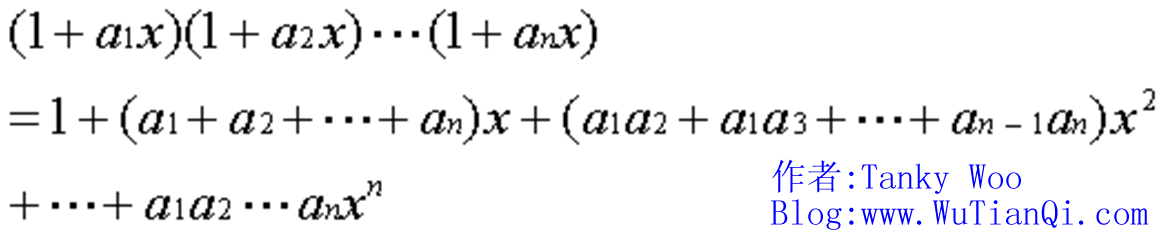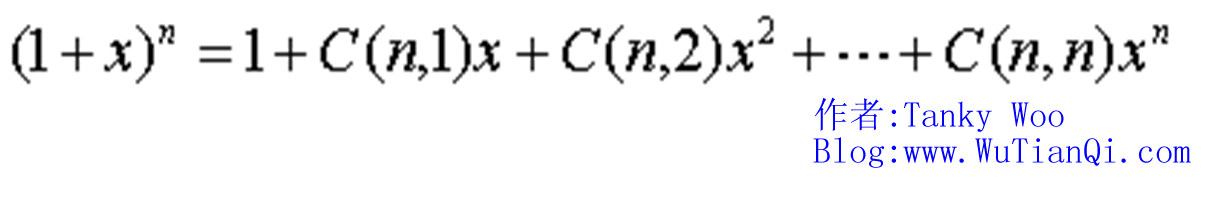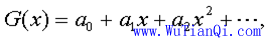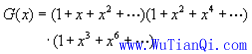# 母函数（Generating function）详解 --- TankyWoo

02 Aug 2010

--- Tanky Woo

> > 1.“把组合问题的加法法则和幂级数的乘幂对应起来” > > > > 2.“母函数的思想很简单 --- 就是把离散数列和幂级数一 一对应起来，把离散数列间的相互结合关系对应成为幂级数间的运算关系，最后由幂级数形式来确定离散数列的构造. “ > >> > 1.x的系数是a1,a2,…an 的单个组合的全体。 > > > > 2. x^2的系数是a1,a2,…a2的两个组合的全体。 > > > > ……… > > > > n. x^n的系数是a1,a2,….an的n个组合的全体（只有1个）。 > >> > 1个1克的砝码可以用函数1+1*x^1表示， > > > > 1个2克的砝码可以用函数1+1*x^2表示， > > > > 1个3克的砝码可以用函数1+1*x^3表示， > > > > 1个4克的砝码可以用函数1+1*x^4表示， > >

1+x^2，也就是1x^0 + 1x^2，也就是上面说的不取2克砝码，此时有1种状态；或者取2克砝码，此时也有1种状态。(分析！)

(1+x)(1+x^2)(1+x^3)(1+x^4)

=(1+x+x^2+x^4)(1+x^3+^4+x^7)

=1 + x + x^2 + 2x^3 + 2x^4 + 2x^5 + 2x^6 + 2*x^7 + x^8 + x^9 + x^10``````#include
using namespace std;
// Author: Tanky Woo
// www.wutianqi.com
const int _max = 10001;
// c1是保存各项质量砝码可以组合的数目
// c2是中间量，保存没一次的情况
int c1[_max], c2[_max];
int main()
{   //int n,i,j,k;
int nNum;   //
int i, j, k;

while(cin >> nNum)
{
for(i=0; i<=nNum; ++i)   // ---- ①
{
c1[i] = 1;
c2[i] = 0;
}
for(i=2; i<=nNum; ++i)   // ----- ②
{

for(j=0; j<=nNum; ++j)   // ----- ③
for(k=0; k+j<=nNum; k+=i)  // ---- ④
{
c2[j+k] += c1[j];
}
for(j=0; j<=nNum; ++j)     // ---- ⑤
{
c1[j] = c2[j];
c2[j] = 0;
}
}
cout << c1[nNum] << endl;
}
return 0;
}
``````

> > ① 、首先对c1初始化，由第一个表达式(1+x+x^2+..x^n)初始化，把质量从0到n的所有砝码都初始化为1. > > > > ② 、 i从2到n遍历，这里i就是指第i个表达式，上面给出的第二种母函数关系式里，每一个括号括起来的就是一个表达式。 > > > > ③、j 从0到n遍历，这里j就是(前面i個表达式累乘的表达式)里第j个变量，(这里感谢一下seagg朋友给我指出的错误，大家可以看下留言处的讨论)。如(1+x)(1+x^2)(1+x^3)，j先指示的是1和x的系数，i=2执行完之后变为 （1+x+x^2+x^3）(1+x^3)，这时候j应该指示的是合并后的第一个括号的四个变量的系数。 > > > > ④ 、 k表示的是第j个指数，所以k每次增i（因为第i个表达式的增量是i）。 > > > > ⑤ 、把c2的值赋给c1,而把c2初始化为0，因为c2每次是从一个表达式中开始的。 > >

（相应题目解析均在相应的代码里分析）

HDOJ：1709，1028、1709、1085、1171、1398、2069、2152

（原创文章，欢迎各位转载，但是请不要任意删除文章中链接，请自觉尊重文章版权，违法必究，谢谢合作。Tanky Woo原创, www.WuTianQi.com）

1.在维基百科里讲到了普通母函數、指數母函數、L級數、貝爾級數和狄利克雷級數：

http://zh.wikipedia.org/zh-tw/%E6%AF%8D%E5%87%BD%E6%95%B0

2．Matrix67大牛那有篇文章：什么是生成函数：

http://www.matrix67.com/blog/archives/120

3.大家可以看看杭电的ACM课件的母函数那篇，我这里的图片以及一些内容都引至那。

Tanky Woo原创文章，转载请注明出处：http://www.wutianqi.com/?p=596

(仅作保留所用，里面有错误，建议看我的最新版本，关注本博客：http://www.wutianqi.com/?p=539)